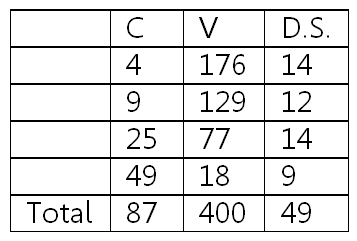### Chapters Whose Number is the Square of a Prime Number

#### الخميس، 23 إبريل 2015 - 1:14There are four chapters in the Quran that have chapter numbers that are squares of primes; namely chapters 4 (2^2), 9 (3^2), 25 (5^2) and 49 (7^2). These chapters exhibit interesting mathematical relationships between their Chapter number, C, their number of verses, V, and the digit sum of the number of verses, D.S. (the sum of the digits that make up the number; e.g. 176 had a digit sum of 1+7+6 = 14):

 D.S. V C 14 176 4 12 129 9 14 77 25 9 18 49 49 400 87 Total

RESULTS

The total number of verses is 400 which is a perfect square: 400 = 20*20.

The total of the digit sums of the chapter numbers is a perfect square: 49 = 7*7.

Digit sum of the total of the number of verses is 4+0+0 = 4 which is a perfect square = 2*2.

The sum of the total number of verses + the total of the chapter numbers is 400+87 = 487 which is additive prime (a prime number whose digit sum, 4+8+7=19, is also a prime).

The sum of the total number of verses + the total of the digit sum of the number of verses is 400+49 = 449 which is also additive prime (its D.S. is 4+4+9=17).

The concatenation of the total number of verses and the total of the chapter numbers, 40087 is also additive prime.

 الأكثر قراءة
الموقع الرسمي حم © 2015, كل الحقوق محفوظة |
powered by
Official Site 7ameem © 2015, All right reserved